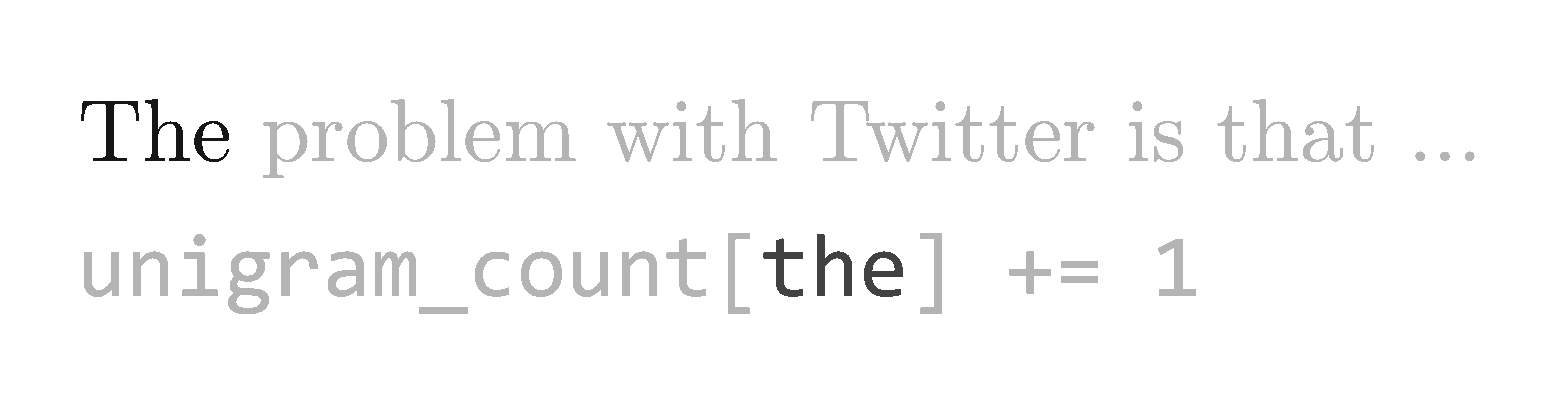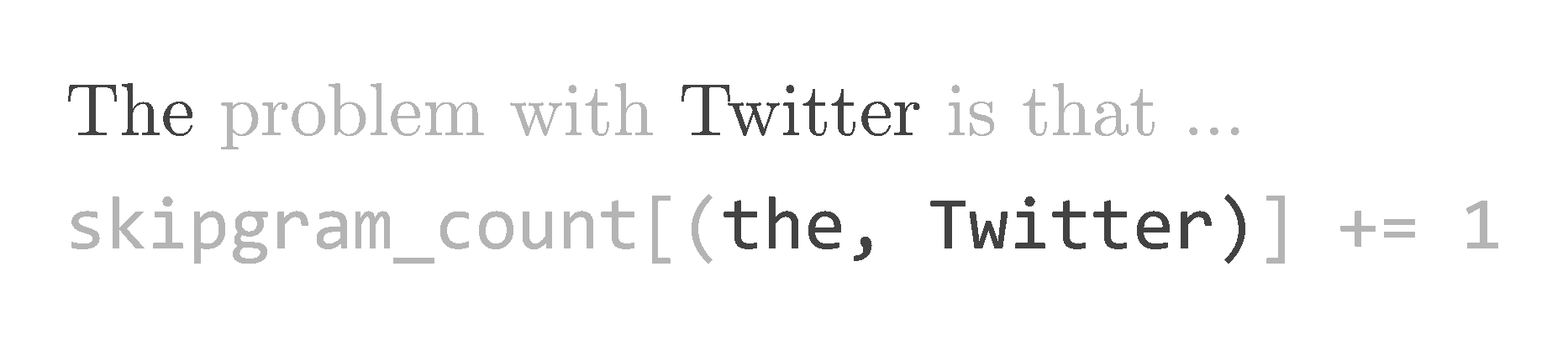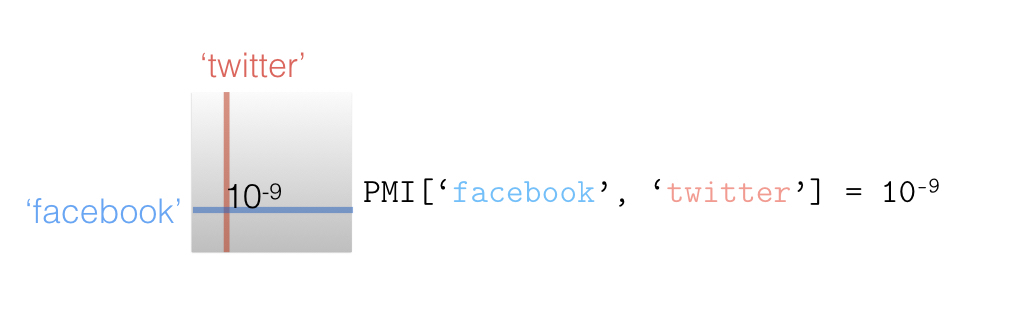# Stop Using word2vec

When I started playing with word2vec four years ago I needed (and luckily had) tons of supercomputer time. But because of advances in our understanding of word2vec, computing word vectors now takes fifteen minutes on a single run-of-the-mill computer with standard numerical libraries1. Word vectors are awesome but you don’t need a neural network – and definitely don’t need deep learning – to find them2. So if you’re using word vectors and aren’t gunning for state of the art or a paper publication then stop using word2vec.

When we’re finished you’ll measure word similarities:

facebook ~ twitter, google, ...

… and the classic word vector operations: zuckerberg - facebook + microsoft ~ nadella

…but you’ll do it mostly by counting words and dividing, no gradients harmed in the making!

## The recipe

Let’s assume you’ve already gotten your hands on the Hacker News corpus and cleaned & tokenized it (or downloaded the preprocessed version here). Here’s the method:

1. Unigram Probability. How often do I see word1 and word2 independently?Example: This is just a simple word count that fills in the unigram_counts array. Then divide unigram_counts array by it’s sum to get the probability p and get numbers like: p('facebook') is 0.001% and p('lambda') is 0.000001%

2. Skipgram Probability. How often did I see word1 nearby word2? These are called ‘skipgrams’ because we can ‘skip’ up to a few words between word1 and word2.3Example: Here we’re counting pairs of words that are near each other, but not necessarily right next to each other. After normalizing the skipgram_count array, you’ll get a measurement of word-near-word probabilities like p('facebook', 'twitter'). If the value for p('facebook', 'twitter') is 10^-9 then out of one billion skipgram tuples you’ll typically see ‘facebook’ and ‘twitter’ once. For another skipgram like p('morning', 'facebook') this fraction might be much larger, like 10^-5, simply because the word morning is a frequent word.

3. Normalized Skipgram Probability (or PMI). Was the skipgram frequency higher or lower than what we expected from the unigram frequency? Some words are extremely common, some are very rare, so divide the skipgram frequency by the two unigram frequencies. If the result is more than 1.0, then that skipgram occurred more frequently than the unigram probabilities of the two input words and we’ll call the two input words “associated”. The greater the ratio, the more associated. For ratios less than 1.0, the more “anti-associated”. If we take the log of this number it’s called the pointwise mutual information (PMI) of word X and word Y. This is a well-understood measurement in the information theory community and represents how frequently X and Y coincide ‘mutually’ (or jointly) rather than independently.Example 1: If we look at the association between facebook and twitter we’ll see that it’s above 1.0: p('facebook', 'twitter') / p('facebook') / p('twitter') = 1000. So facebook and twitter have unusually high-cooccurrence and we infer that they must be associated or similar. Note that we haven’t done any neural network stuff and no math aside from counting & dividing, but we can already measure how associated two words are. Later on we’ll calculate word vectors from this data, and those vectors will be constrained to reproduce these word-to-word relationships. 4

Example 2: For facebook and okra the PMI (p('facebook', 'okra') / p('facebook') / p('okra')) is close to 1.0, so facebook and okra aren’t associated empirically in the data. Later on we’ll form vectors that reconstruct and respect this relationship, and so will have little overlap. But of course the word counts are noisy, and this noise induces spurious associations between words.

4. PMI Matrix. Make a big matrix where each row represents word X and each column represents word Y and each value is the PMI we calculated in step 3: PMI(X, Y) = log (p(x, y) / p(x) / p(y)). Because we have as many rows and columns as we have words, the size of the matrix is (n_vocabulary, n_vocabulary). And because n_vocabulary is typically 10k-100k, this is a big matrix with lots of zeros, so it’s best to use sparse array data structures to represent the PMI matrix.5. SVD. Now we reduce the dimensionality of that matrix. This effectively compresses our giant matrix into two smaller matrices. Each of these smaller matrices form a set of word vectors with size (n_vocabulary, n_dim).5 Each row of one matrix represents a single word vector. This is a straightforward operation in any linear algebra library, and in Python it looks like: U, S, V = scipy.sparse.linalg.svds(PMI, k=256)Example. The SVD is one of the most fundamental and beautiful tools you can use in machine learning and is what’s doing most of the magic. Read more about it in Jeremy Kun’s excellent series. Here, we can think of it as compressing the original input matrix which (counting zeros) had close to ~10 billion entries (100k x 100k) reduced into two matrices with a total of ~50 million entries (100k x 256 x 2), a 200x reduction in space. The SVD outputs a space that is orthogonal, which is where we get our “linear regularity” and is where we get the property of being able to add and subtract word vectors meaningfully.

6. Searching. Each row of the SVD output matrices is a word vector. Once you have these word eigenvectors, you can search for the tokens closest to consolas, which is a font popular in programming.

# In psuedocode:
# Get the row vector corresponding to the word 'consolas'
vector_consolas = U['consolas']
# Get how similar it is to all other words
similarities = dot(U, vector_consolas)
# Sort by similarity, and pick the most similar
most_similar = tokens[argmax(similarities)]
most_similar


So searching for consolas yields verdana and inconsolata as most similar – which makes sense as these are other fonts. Searching for Functional Programming yields FP (an acronym), Haskell (a popular functional language), and OOP (which stands for Object Oriented Programming, an alternative to functional programming). Further, adding and subtracting these vectors and then searching gets word2vec’s hallmark feature: in computing the analogy Mark Zuckerberg - Facebook + Amazon we can relate the CEO of Facebook to that of Amazon, which appropriately evaluates to the Jeff Bezos token.

It’s a hell of a lot more intuitive & easier to count skipgrams, divide by the word counts to get how ‘associated’ two words are and SVD the result than it is to understand what even a simple neural network is doing.

So stop using the neural network formulation, but still have fun making word vectors!

### Footnotes## Come Work with Us!

We’re a diverse team dedicated to building great products, and we’d love your help. Do you want to build amazing products with amazing peers? Join us!